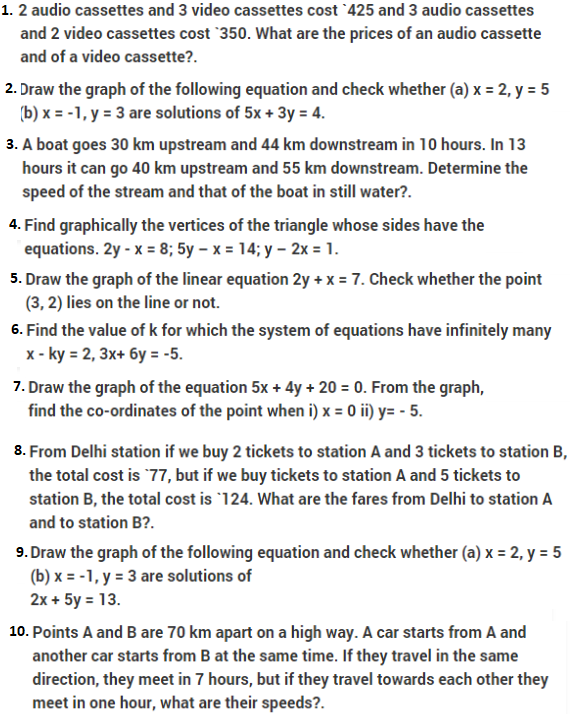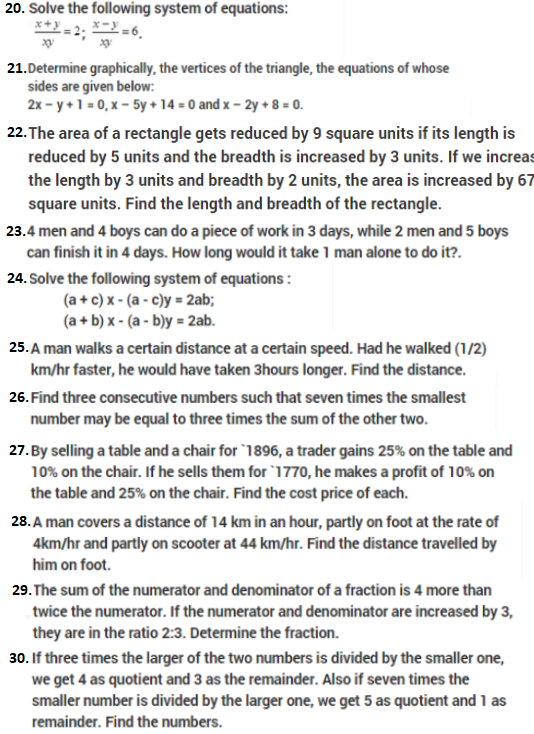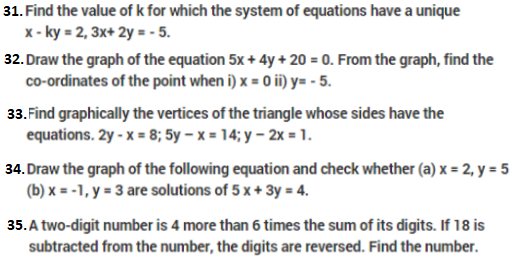# Important Questions Class 10 Maths Chapter 3 Linear Equations In Two Variables

Important class 10 maths questions for Chapter 3 Linear Equations In Two Variables are given here for practice. These questions will help the CBSE class 10 students to have a better understanding of the linear equations In two variables concept in NCERT maths. These class 10 chapter 10 questions are also important for CBSE class 10 Maths exam.

Also Check:

## Important Linear Equations In Two Variables Questions For Class 10 (Chapter 3)